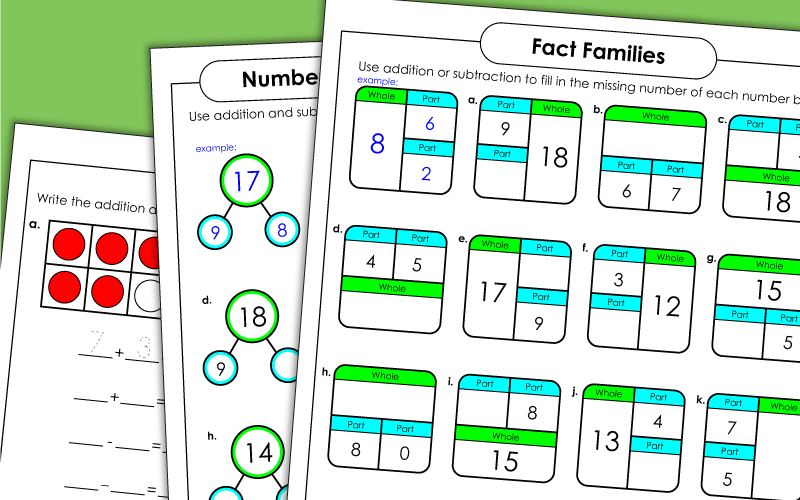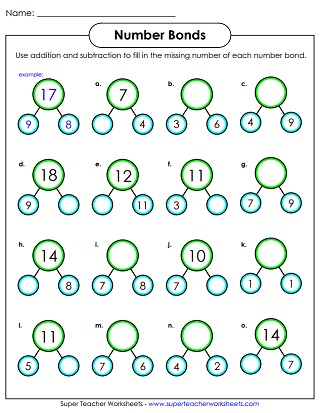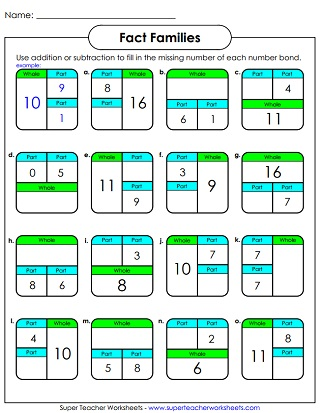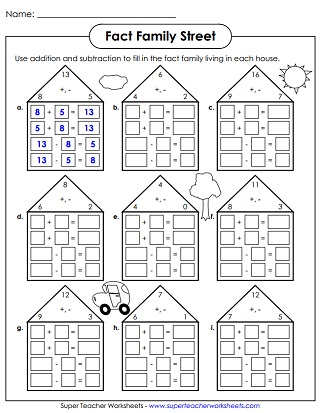# Fact Families / Number Families (Addition Subtraction)

Here are some printable number family and number bond worksheets to show the relationships between addition and subtraction problems.## Fact Family Flashcards

Here's a complete set of addition and subtraction fact family flashcards.

## Fact Family Triangles

Write the correct numbers in each addition/subtraction fact family triangle.
More fact family subtraction-addition fact family triangles to solve.
Write the correct addition/subtraction fact family for each set of numbers.
Another worksheet with fact family houses in which students much write the correct numbers.

## Fact Family Boxes

Use addition or subtraction to determine the missing number in each part-part-whole box.

## Ten Frame Fact Families

Complete the number family shown by each ten frame. All sums are 10 or less.
Complete the number family shown by each pair of ten frames. All sums and minuends are 20 or less.

## Number Bonds

Add or subtract to find the missing part of each number bond.
Complete twelve snowman number bond by filling in the missing number(s).

Basic Subtraction Worksheets

Worksheets with basic subtraction facts

Multiplication and Division Fact Families

Division and multiplication fact family problems and number bonds

## Sample Worksheet ImagesMy Account
Site Information